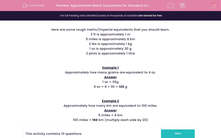# Approximate Metric Equivalents for Standard Imperial Measures

In this worksheet, students revise changing imperial units to their approximate metric equivalents.Key stage:  KS 2

Curriculum topic:   Maths and Numerical Reasoning

Curriculum subtopic:   Metric & Imperial

Difficulty level:#### Worksheet Overview

Here are some rough metric/imperial equivalents that you should learn.

3 ft is approximately 1 m
5 miles is approximately 8 km
2 lbs is approximately 1 kg
1 oz is approximately 30 g
2 pints is approximately 1 litre

Example 1
Approximately how many grams are equivalent to 4 oz.

1 oz = 30g
4 oz = 4 × 30 = 120 g

Example 2
Approximately how many km are equivalent to 100 miles.